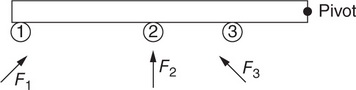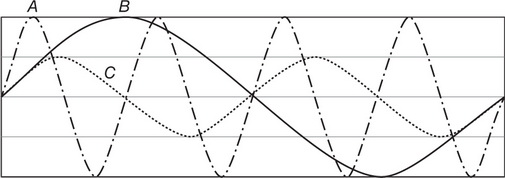# AP Physics 1 Practice Test 14

### Test Information10 questions18 minutes

1. It is known that a lab cart is moving east at 25 cm/s at time t1 = 0.10 s, and then moving east at 15 cm/s at t2 = 0.20 s. Is this enough information to determine the direction of the net force acting on the cart between t1 and t2?

2.A rigid rod is pivoted at its right end. Three forces of identical magnitude but different directions are applied at the positions 1, 2, and 3 as shown. Which of the following correctly ranks the torques τ1, τ2, and τ3 provided by the forces F1, F2, and F3?

3. A block hanging vertically from a spring undergoes simple harmonic motion. Which of the following graphs could represent the acceleration a as a function of position x for this block, where x = 0 is the midpoint of the harmonic motion?

4.The preceding diagram represents a photograph of three transverse waves, each of which is moving to the right in the same material as the others. Which of the following ranks the waves by their amplitudes?

5. The mass of the Earth is 5.97 × 1024 kg. The Moon, whose center is 3.84 × 108 m from the Earth’s center, has mass 7.35 × 1022 kg. Which of the following is the best estimate of the gravitational force of the Earth on the Moon?

6. A children’s toy consists of a cart whose very light wheels are attached to a rubber band. This rubber band can wind and unwind around the axle supporting the wheels.

This toy is given a shove, after which the toy rolls across a flat surface and up a ramp. It is observed that the toy does not go a consistent distance up the ramp—in some trials it ends up higher than in other trials, even though the shove imparts the same kinetic energy to the cart each time. Which of the following is a reasonable explanation for this phenomenon?

7. A man stands on a platform scale in an elevator. The elevator moves upward, speeding up. What is the action-reaction force pair to the man’s weight?

8.The preceding diagram shows a speaker mounted on a cart that moves to the right at constant speed v. Wave fronts for the constant-frequency sound wave produced by the speaker are indicated schematically in the diagram. Which of the following could represent the wave fronts produced by the stationary speaker playing the same note?

9. A table supports a wooden block placed on the tabletop. Which fundamental force of nature is responsible for this interaction, and why?

10. A solid sphere (I = 0.06 kg·m2) spins freely around an axis through its center at an angular speed of 20 rad/s. It is desired to bring the sphere to rest by applying a friction force of magnitude 2.0 N to the sphere’s outer surface, a distance of 0.30 m from the sphere’s center. How much time will it take the sphere to come to rest?